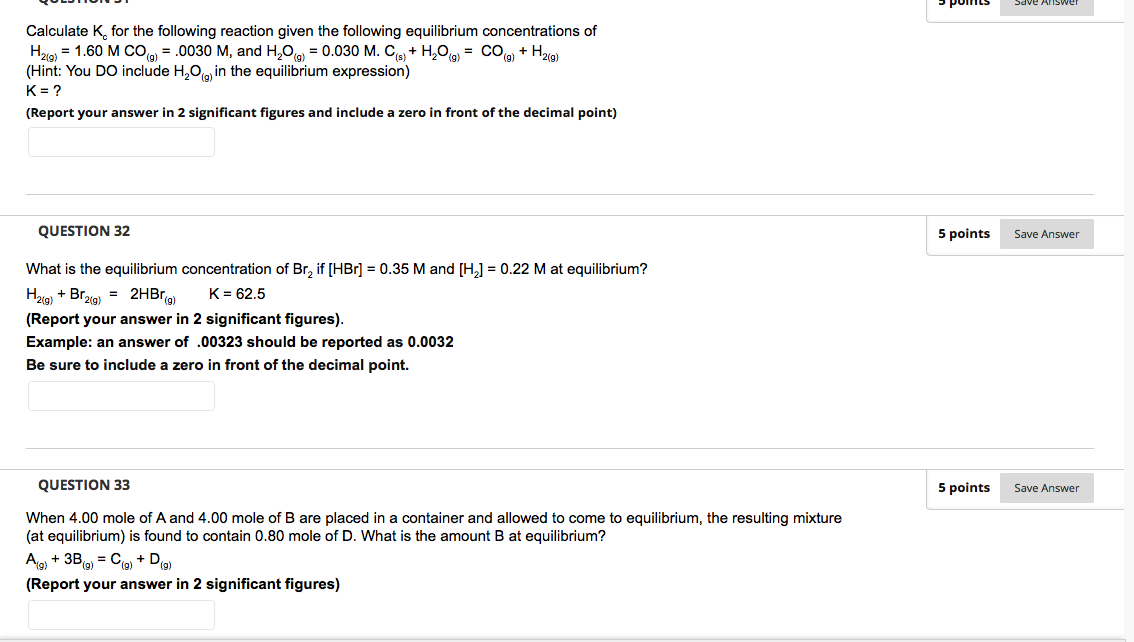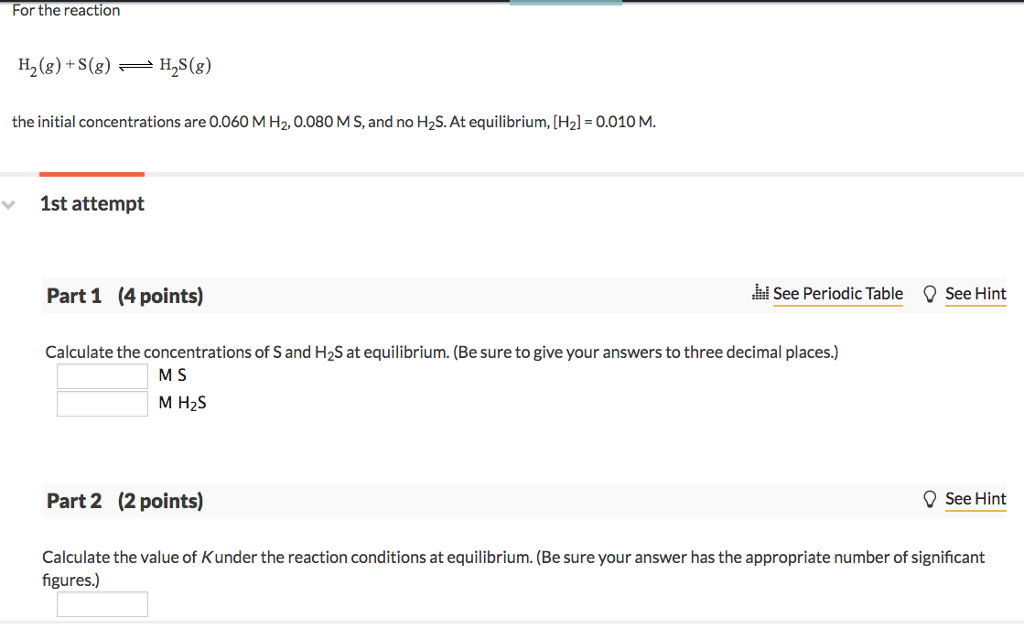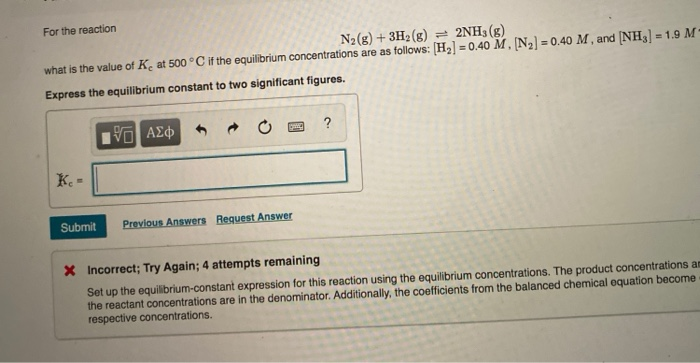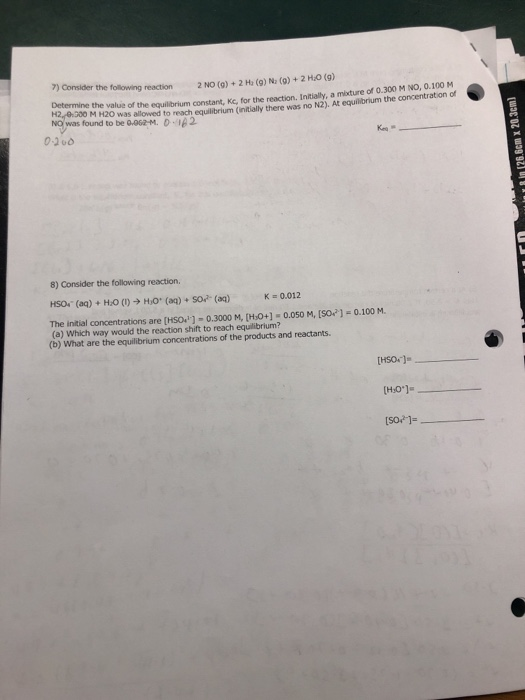Question

# For the unbalanced reaction H2​(g)+NO(g) ---> H2​O(g)+N2​(g): the initial concentrations are 5.25 M H2, 9.00 M...

For the unbalanced reaction H2​(g)+NO(g) ---> H2​O(g)+N2​(g):

the initial concentrations are 5.25 M H2, 9.00 M NO, and no H2O or N2. At equilibrium, [N2] = 1.75 M.

A) Balance the equation using lowest-whole-number coefficients. Be sure to include states of matter in your equation.

B) Calculate the value of K under the reaction conditions at equilibrium.

H2(g) + NO(g) ----------- H2O(g) + N2(g)

The balanced equation i s

2 H2(g) + 2NO(g) ----------- 2H2O(g) + N2(g)

Initial concentration of H2= 5.25M

[NO] = 9.00M

at equilibrium [N2] = 1.75M

a)

The balanced equation is

2 H2(g) + 2NO(g) ----------- 2H2O(g) + N2(g)

B)

2 H2(g) + 2NO(g) ----------- 2H2O(g) + N2(g)

Initial                               5.25         9.00                    0               0

chnage                            -2x           -2x                     +2x             +x

equilibrium                  5.25-2x        9.00-2x               +2x               +x ( 1.75)

according to given data

at equilibrium [N2] = x= 1.75M

x= 1.75M

at equilibrium

[H2] = 5.25 - 2x = 5.25 - 2(1.75) = 1.75M

[NO] = 9.00-2x= 9.00 - 2(1.75) = 5.5M

[H2O] = 2x = 2 x1.75 = 3.5M

K= [H2O]^2 [N2]/ [H2[^2[NO]^2

K = ( 3.5)^2 x 1.75 / (1.75)^2 x(5.5)^2

K= 21.4375/92.641

K = 0.231

Equilibrium constant = K = 0.231.

#### Earn Coins

Coins can be redeemed for fabulous gifts.

Similar Homework Help Questions
• ### Calculate Kc for the following reaction given the following equilibrium concentrations of H2(g) = 1.60 M...Calculate Kc for the following reaction given the following equilibrium concentrations of H2(g) = 1.60 M CO(g) = .0030 M, and H2O(g) = 0.030 M. C(s) + H2O(g) =  CO(g) + H2(g) (Hint: You DO include H2O(g) in the equilibrium expression) K = ? Save Answer Calculate K, for the following reaction given the following equilibrium concentrations of H2(9) = 1.60 M COO) = .0030 M, and H,00 = 0.030 M. Co+H,09 = COO + H2(9) (Hint: You Do include H,0...

• ### For the reaction H_2(g) + S(g) H_2S(g) the initial concentrations are 0.060 M H_2, 0.080 MS,...For the reaction H_2(g) + S(g) H_2S(g) the initial concentrations are 0.060 M H_2, 0.080 MS, and no H_2S. At equilibrium, [H_2] = 0.010 M. Calculate the concentrations of S and H_2S at equilibrium. (Be sure to give your answers to three decimal places.) M S M H_2 S Calculate the value of K under the reaction conditions at equilibrium. (Be sure your answer has the appropriate number of significant figures.)

• ### For the reaction N2(g) + 3H2(g) = 2NH3(g) what is the value of Ke at 500°C if the equilibrium concentrations...For the reaction N2(g) + 3H2(g) = 2NH3(g) what is the value of Ke at 500°C if the equilibrium concentrations are as follows: [H2] = 0.40 M, (N2) = 0.40 M, and (NH3) = 1.9 M Express the equilibrium constant to two significant figures. V AE OE ? Submit Previous Answers Request Answer X Incorrect; Try Again; 4 attempts remaining Set up the equilibrium-constant expression for this reaction using the equilibrium concentrations. The product concentrations a the reactant concentrations are...

• ### 1. The initial concentrations of reactants and products for this reaction are given below. N2(g) +...

1. The initial concentrations of reactants and products for this reaction are given below. N2(g) + O2(g) ⇄ 2NO(g) Calculate Q for this reaction. Answer this to one decimal place (e.g. 10.2) The initial concentration of N2 is 1.0 M The initial concentration of O2 is 1.0 M The initial concentration of NO is 2.5 M 2. The reaction below is not at equilibria and Q = 1.7. The equilibrium constant is K = 0.230. 2SO3(g) ⇌ 2SO2(g) + O2(g)...

• ### Consider the following reaction: N2(g)+3H2(g)⇌2NH3(g) Complete the following table. Assume that all concentrations are equilibrium concentrations...

Consider the following reaction: N2(g)+3H2(g)⇌2NH3(g) Complete the following table. Assume that all concentrations are equilibrium concentrations in M. T (K) [N2] [H2] [NH3] [Kc] 500 0.115 0.105 0.439 − 575 0.105 M − 0.128 M 9.6 775 0.130 M 0.145 M − 0.0584 Find Kc at 500 K. Find [H2] at 575 K. Find [NH3] at 775 K.

• ### Calculate the equilibrium concentrations of H2, I2, and HI at 700 K if the initial concentrations...

Calculate the equilibrium concentrations of H2, I2, and HI at 700 K if the initial concentrations are [H2] = 0.200 M and [I2] = 0.400 M. The equilibrium constant Kc for the reaction following reaction is 57.0 at 700 K. (Show Work) H2(g)+I2(g)<---                    ---->2HI(g)

• ### 1. The initial concentrations of reactants and products for this reaction are given below. N2(g)   +  ...

1. The initial concentrations of reactants and products for this reaction are given below. N2(g)   +   O2(g)  ⇄ 2NO(g) Calculate Q for this reaction. Answer this to one decimal place (e.g. 10.2) The initial concentration of N2 is 1.0 M The initial concentration of O2 is 1.0 M The initial concentration of NO is 2.5 M 2. Calculate the equilibrium concentration of Cl2 in that results from the decomposition of COCl2 with an initial concentration of 0.3166 M. COCl2(g) ⇌ CO(g)...

• ### The reaction for the formation of ammonia is shown as: N2(g) + 3 H2(g) ⇄ 2...

The reaction for the formation of ammonia is shown as: N2(g) + 3 H2(g) ⇄ 2 NH3(g)       Kc=? Write the equilibrium constant expression (Kc) for this reaction. Calculate the value of Kc at 500. K for the formation of ammonia in part a) using the following measured concentrations for the equilibrium mixture: [N2] = 3.0 x 10-­‐2  M; [H2] = 3.7 x 10-­‐2  M; [NH3] = 1.6 x 10-­‐2  M.                                                                                [1.7 x 102] Now, calculate the value of Kc for the formation of...

• ### 2.1 For the reaction N2(g) + C2H2(g) == 2HCN(g) K=0.0324 What are the equilibrium concentrations of...

2.1 For the reaction N2(g) + C2H2(g) == 2HCN(g) K=0.0324 What are the equilibrium concentrations of all species present if the initial concentrations are [N2] = [C2H2] = [HCN] = 2.50 M?

• ### The following reaction 2NO(0) + 2H2 (g) № (g) + 2 HaO (g) H2/0000 M quilbrium constant, Ke, for t...the following reaction 2NO(0) + 2H2 (g) № (g) + 2 HaO (g) H2/0000 M quilbrium constant, Ke, for the reaction. Initially, a mixture of 0.300 M NO, 0.100 M NOwas found to beg,062-м. D.ig2 the value of the brium (init h N2)t eubrhum the conentreton dr H20 was allowed to reach equilibrium ( 0-200 8) Consider the following reaction. HSO.. (aq) + H2O (I) → HO. (aq) + SO2-(aq) The initial concentrations are [HSO門-0.3000 M, [HO+]s 0.050 M. [SO...